CUET  >  Mirror Images Quiz I, Non Verbal Reasoning

# Mirror Images Quiz I, Non Verbal Reasoning - CUET

Test Description

## 25 Questions MCQ Test General Test Preparation for CUET - Mirror Images Quiz I, Non Verbal Reasoning

Mirror Images Quiz I, Non Verbal Reasoning for CUET 2023 is part of General Test Preparation for CUET preparation. The Mirror Images Quiz I, Non Verbal Reasoning questions and answers have been prepared according to the CUET exam syllabus.The Mirror Images Quiz I, Non Verbal Reasoning MCQs are made for CUET 2023 Exam. Find important definitions, questions, notes, meanings, examples, exercises, MCQs and online tests for Mirror Images Quiz I, Non Verbal Reasoning below.
Solutions of Mirror Images Quiz I, Non Verbal Reasoning questions in English are available as part of our General Test Preparation for CUET for CUET & Mirror Images Quiz I, Non Verbal Reasoning solutions in Hindi for General Test Preparation for CUET course. Download more important topics, notes, lectures and mock test series for CUET Exam by signing up for free. Attempt Mirror Images Quiz I, Non Verbal Reasoning | 25 questions in 50 minutes | Mock test for CUET preparation | Free important questions MCQ to study General Test Preparation for CUET for CUET Exam | Download free PDF with solutions
 1 Crore+ students have signed up on EduRev. Have you?
Mirror Images Quiz I, Non Verbal Reasoning - Question 1

### Directions to Solve In each of the following questions you are given a combination of alphabets and/or numbers followed by four alternatives (1), (2), (3) and (4). Choose the alternative which is closely resembles the mirror image of the given combination. Question - Choose the alternative which is closely resembles the mirror image of the given combination.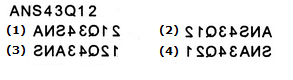Mirror Images Quiz I, Non Verbal Reasoning - Question 2

### Directions to Solve In each of the following questions you are given a combination of alphabets and/or numbers followed by four alternatives (1), (2), (3) and (4). Choose the alternative which is closely resembles the mirror image of the given combination. Question - Choose the alternative which is closely resembles the mirror image of the given combination.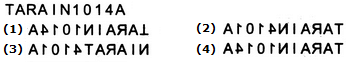Mirror Images Quiz I, Non Verbal Reasoning - Question 3

### Directions to Solve In each of the following questions you are given a combination of alphabets and/or numbers followed by four alternatives (1), (2), (3) and (4). Choose the alternative which is closely resembles the mirror image of the given combination. Question - Choose the alternative which is closely resembles the mirror image of the given combination.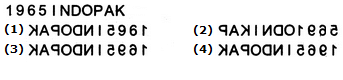Mirror Images Quiz I, Non Verbal Reasoning - Question 4

In each of the following questions you are given a combination of alphabets and/or numbers followed by four alternatives (1), (2), (3) and (4). Choose the alternative which is closely resembles the mirror image of the given combination.

Question -

Choose the alternative which is closely resembles the mirror image of the given combination.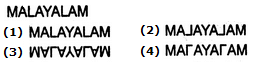Mirror Images Quiz I, Non Verbal Reasoning - Question 5

5.Choose the alternative which is closely resembles the mirror image of the given combination.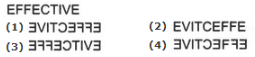Mirror Images Quiz I, Non Verbal Reasoning - Question 6

In each of the following questions you are given a combination of alphabets and/or numbers followed by four alternatives (1), (2), (3) and (4). Choose the alternative which is closely resembles the mirror image of the given combination.

Question -

Choose the alternative which is closely resembles the mirror image of the given combination.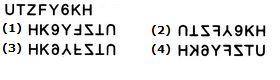Mirror Images Quiz I, Non Verbal Reasoning - Question 7

In each of the following questions you are given a combination of alphabets and/or numbers followed by four alternatives (1), (2), (3) and (4). Choose the alternative which is closely resembles the mirror image of the given combination.

Question -

Choose the alternative which is closely resembles the mirror image of the given combination.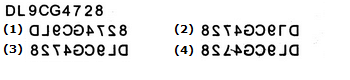Mirror Images Quiz I, Non Verbal Reasoning - Question 8

Directions to Solve

In each of the following questions you are given a combination of alphabets and/or numbers followed by four alternatives (1), (2), (3) and (4). Choose the alternative which is closely resembles the mirror image of the given combination.

Question -

Choose the alternative which is closely resembles the mirror image of the given combination.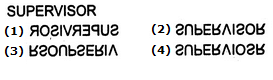Detailed Solution for Mirror Images Quiz I, Non Verbal Reasoning - Question 8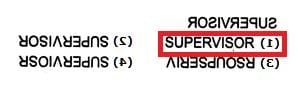Mirror Images Quiz I, Non Verbal Reasoning - Question 9

In each of the following questions you are given a combination of alphabets and/or numbers followed by four alternatives (1), (2), (3) and (4). Choose the alternative which is closely resembles the mirror image of the given combination.

Question -

Choose the alternative which is closely resembles the mirror image of the given combination.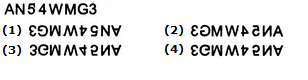Mirror Images Quiz I, Non Verbal Reasoning - Question 10

In each of the following questions you are given a combination of alphabets and/or numbers followed by four alternatives (1), (2), (3) and (4). Choose the alternative which is closely resembles the mirror image of the given combination.

Question -

Choose the alternative which is closely resembles the mirror image of the given combination.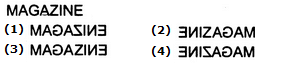Mirror Images Quiz I, Non Verbal Reasoning - Question 11

In each of the following questions you are given a combination of alphabets and/or numbers followed by four alternatives (1), (2), (3) and (4). Choose the alternative which is closely resembles the mirror image of the given combination.

Question -

Choose the alternative which is closely resembles the mirror image of the given combination.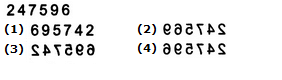Mirror Images Quiz I, Non Verbal Reasoning - Question 12

In each of the following questions you are given a combination of alphabets and/or numbers followed by four alternatives (1), (2), (3) and (4). Choose the alternative which is closely resembles the mirror image of the given combination.

Question -

Choose the alternative which is closely resembles the mirror image of the given combination.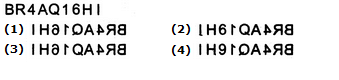Mirror Images Quiz I, Non Verbal Reasoning - Question 13

In each of the following questions you are given a combination of alphabets and/or numbers followed by four alternatives (1), (2), (3) and (4). Choose the alternative which is closely resembles the mirror image of the given combination.

Question -

Choose the alternative which is closely resembles the mirror image of the given combination.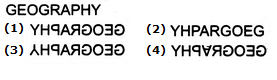Mirror Images Quiz I, Non Verbal Reasoning - Question 14

In each of the following questions you are given a combination of alphabets and/or numbers followed by four alternatives (1), (2), (3) and (4). Choose the alternative which is closely resembles the mirror image of the given combination.

Question -

Choose the alternative which is closely resembles the mirror image of the given combination.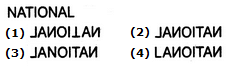Mirror Images Quiz I, Non Verbal Reasoning - Question 15

In each of the following questions you are given a combination of alphabets and/or numbers followed by four alternatives (1), (2), (3) and (4). Choose the alternative which is closely resembles the mirror image of the given combination.

Question -

Choose the alternative which is closely resembles the mirror image of the given combination.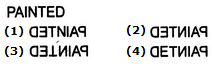Mirror Images Quiz I, Non Verbal Reasoning - Question 16

In each of the following questions you are given a combination of alphabets and/or numbers followed by four alternatives (1), (2), (3) and (4). Choose the alternative which is closely resembles the mirror image of the given combination.

Question -

Choose the alternative which is closely resembles the mirror image of the given combination.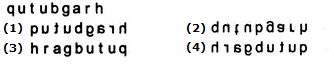Mirror Images Quiz I, Non Verbal Reasoning - Question 17

In each of the following questions you are given a combination of alphabets and/or numbers followed by four alternatives (1), (2), (3) and (4). Choose the alternative which is closely resembles the mirror image of the given combination.

Question -

Choose the alternative which is closely resembles the mirror image of the given combination.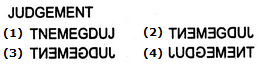Mirror Images Quiz I, Non Verbal Reasoning - Question 18

In each of the following questions you are given a combination of alphabets and/or numbers followed by four alternatives (1), (2), (3) and (4). Choose the alternative which is closely resembles the mirror image of the given combination.

Question -

Choose the alternative which is closely resembles the mirror image of the given combination.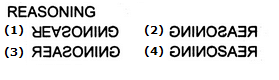Mirror Images Quiz I, Non Verbal Reasoning - Question 19

In each of the following questions you are given a combination of alphabets and/or numbers followed by four alternatives (1), (2), (3) and (4). Choose the alternative which is closely resembles the mirror image of the given combination.

Question -

Choose the alternative which is closely resembles the mirror image of the given combination.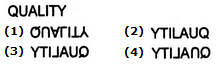Mirror Images Quiz I, Non Verbal Reasoning - Question 20

Directions to Solve

In each of the following questions you are given a combination of alphabets and/or numbers followed by four alternatives (1), (2), (3) and (4). Choose the alternative which is closely resembles the mirror image of the given combination.

Question -

Choose the alternative which is closely resembles the mirror image of the given combination.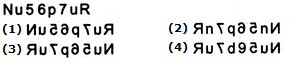Detailed Solution for Mirror Images Quiz I, Non Verbal Reasoning - Question 20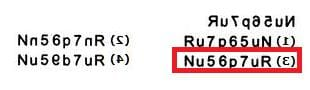Mirror Images Quiz I, Non Verbal Reasoning - Question 21

Directions to Solve

In each of the following questions you are given a combination of alphabets and/or numbers followed by four alternatives (1), (2), (3) and (4). Choose the alternative which is closely resembles the mirror image of the given combination.

Question -

Choose the alternative which is closely resembles the mirror image of the given combination.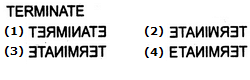Detailed Solution for Mirror Images Quiz I, Non Verbal Reasoning - Question 21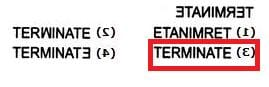Mirror Images Quiz I, Non Verbal Reasoning - Question 22

In each of the following questions you are given a combination of alphabets and/or numbers followed by four alternatives (1), (2), (3) and (4). Choose the alternative which is closely resembles the mirror image of the given combination.

Question -

Choose the alternative which is closely resembles the mirror image of the given combination.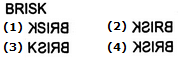Mirror Images Quiz I, Non Verbal Reasoning - Question 23

Directions to Solve

In each of the following questions you are given a combination of alphabets and/or numbers followed by four alternatives (1), (2), (3) and (4). Choose the alternative which is closely resembles the mirror image of the given combination.

Question -

Choose the alternative which is closely resembles the mirror image of the given combination.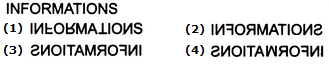Detailed Solution for Mirror Images Quiz I, Non Verbal Reasoning - Question 23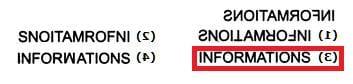Mirror Images Quiz I, Non Verbal Reasoning - Question 24

In each of the following questions you are given a combination of alphabets and/or numbers followed by four alternatives (1), (2), (3) and (4). Choose the alternative which is closely resembles the mirror image of the given combination.

Question -

Choose the alternative which is closely resembles the mirror image of the given combination.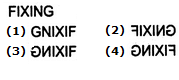Mirror Images Quiz I, Non Verbal Reasoning - Question 25

In each of the following questions you are given a combination of alphabets and/or numbers followed by four alternatives (1), (2), (3) and (4). Choose the alternative which is closely resembles the mirror image of the given combination.

Question -

Choose the alternative which is closely resembles the mirror image of the given combination.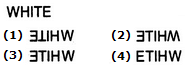## General Test Preparation for CUET

176 videos|328 docs|399 tests
Information about Mirror Images Quiz I, Non Verbal Reasoning Page
In this test you can find the Exam questions for Mirror Images Quiz I, Non Verbal Reasoning solved & explained in the simplest way possible. Besides giving Questions and answers for Mirror Images Quiz I, Non Verbal Reasoning, EduRev gives you an ample number of Online tests for practice

## General Test Preparation for CUET

176 videos|328 docs|399 tests